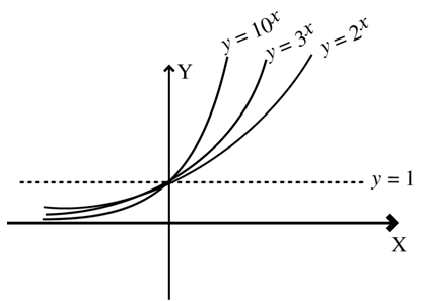# Exponential Functions

For any number a > 0, a ≠ 1, the function f : R → R defined by f(x) = ax is called an exponential function.

For exponential function, the range is always R+ (the set of all positive real numbers).

### Graph of Exponential Function

f(x) = 1 when x = 0. Thus the graph cuts the y axis at y = 1.

For any real value of x, it never becomes zero. Hence the corresponding curves to the above functions do not meet the x-axis for real x.